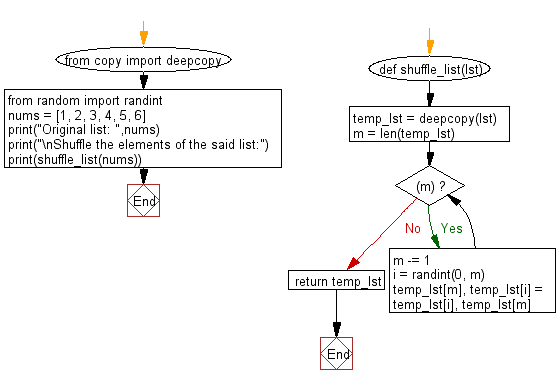﻿ Python: Randomize the order of the values of an list - w3resource# Python: Randomize the order of the values of an list

## Python List: Exercise - 221 with Solution

Write a Python program to randomize the order of the values of an list, returning a new list.

• Uses the Fisher-Yates algorithm to reorder the elements of the list.
• random.shuffle provides similar functionality to this snippet.

From Wikipedia,
The Fisher–Yates shuffle is an algorithm for generating a random permutation of a finite sequence—in plain terms, the algorithm shuffles the sequence. The algorithm effectively puts all the elements into a hat; it continually determines the next element by randomly drawing an element from the hat until no elements remain. The algorithm produces an unbiased permutation: every permutation is equally likely. The modern version of the algorithm is efficient: it takes time proportional to the number of items being shuffled and shuffles them in place.

Sample Solution:

Python Code:

``````from copy import deepcopy
from random import randint
def shuffle_list(lst):
temp_lst = deepcopy(lst)
m = len(temp_lst)
while (m):
m -= 1
i = randint(0, m)
temp_lst[m], temp_lst[i] = temp_lst[i], temp_lst[m]
return temp_lst
nums = [1, 2, 3, 4, 5, 6]
print("Original list: ",nums)
print("\nShuffle the elements of the said list:")
print(shuffle_list(nums))
```
```

Sample Output:

```Original list:  [1, 2, 3, 4, 5, 6]

Shuffle the elements of the said list:
[3, 2, 4, 1, 6, 5]
```

Flowchart:## Visualize Python code execution:

The following tool visualize what the computer is doing step-by-step as it executes the said program:

Python Code Editor:

Have another way to solve this solution? Contribute your code (and comments) through Disqus.

What is the difficulty level of this exercise?

Test your Python skills with w3resource's quiz

﻿

## Python: Tips of the Day

Floor Division:

When we speak of division we normally mean (/) float division operator, this will give a precise result in float format with decimals.

For a rounded integer result there is (//) floor division operator in Python. Floor division will only give integer results that are round numbers.

```print(1000 // 300)
print(1000 / 300)```

Output:

```3
3.3333333333333335```